﻿ Performance of CHMA System Using Orthogonal Complementary Codes under Diversity Incorporated Fading Channels
Journal of Telecommunications System & Management
All submissions of the EM system will be redirected to Online Manuscript Submission System. Authors are requested to submit articles directly to Online Manuscript Submission System of respective journal.
• Research Article
• J Telecommun Syst Manage 2016, Vol 5(2): 136
• DOI: 10.4172/2167-0919.1000136

# Performance of CHMA System Using Orthogonal Complementary Codes under Diversity Incorporated Fading Channels

Judson D1*, Bhaskar V2 and Albert Raj A3
1Department of Electronics and Communication Engineering, St. Xavier’s Catholic College of Engineering, Chunkankadai, Tamilnadu, India
2Department of Electrical and Computer Engineering, San Francisco State University, 1600 Holloway Avenue, CA 94132, USA
3Department of Electronics and Communication Engineering, DMI Engineering College, Aralvaimozhil, Tamilnadu, India
*Corresponding Author: Judson D, Department of Electronics and Communication Engineering, St. Xavier’s Catholic College of Engineering, Chunkankadai-629003, Tamilnadu, India, Tel: 4652232560, Email: [email protected]

Received Date: Jul 15, 2016 / Accepted Date: Aug 04, 2016 / Published Date: Aug 15, 2016

### Abstract

Code-Hopping Multiple Access (CHMA) has been an emerging Multiple Access (MA) technology which offers excellent performance in multipath fading channels with Orthogonal Complementary Codes (OCC). Orthogonality among users is not preserved in multipath channel conditions using the existing orthogonal and semi-orthogonal codes. Further, Multiple Access Interference (MAI) and Multipath Interference (MPI) destroy orthogonality of the code set which in turn increases the error rate during the detection process. An OCC set possesses both ideal auto-correlation and ideal cross-correlation properties for any two codes in the same set. Here, we employ OCC for spreading and prove that the performance of OCC is superior to those of Gold codes in multipath channels where orthogonality does not hold. The error probability expressions for Rayleigh and Nakagami-m fading channels using Maximal Ratio Combining (MRC) are derived for CHMA system using OCCs. We then evaluate the system performance using the proposed technique and show that the new scheme is much better compared to traditional CHMA schemes.

Keywords: Code hopping multiple access; Orthogonal complementary codes; Maximal ratio combining; Rayleigh fading; Nakagami-m fading

#### Introduction

In a limited wireless spectrum, Multiple Access (MA) techniques are required to share the same wireless channel among several users as effectively as possible. MA techniques such as Frequency Division Multiple Access (FDMA), Time Division Multiple Access (TDMA), and Code Division Multiple Access (CDMA) are commonly used to share the same channel among multiple users.

Direct Sequence CDMA (DS-CDMA) supports variable and high data rate transmissions , and hence is adopted in 3G wireless systems. In CDMA, each user suffers from multipath and Multiple Access Interference (MAI), which limit the system capacity and maximum data rate that can be supported over an available bandwidth. MAI is reduced using spreading codes with very low cross-correlation, maintaining power control on each transmitter, employing antenna diversity, and performing multiuser detection . Several multiuser detectors have been introduced  to provide robust demodulation of the transmitted bits in the presence of MAI.

In CDMA, a unique signature code is used for each user, and hence to support a large number of users, the set size of the signature code increases. Orthogonal Code Hopping Multiplexing (OCHM)  was proposed to accommodate more orthogonal downlink channels than the number of orthogonal code words. Although Code Hopping Multiple Access (CHMA) was proposed to accommodate more users than the available number of code words, this resulted in collisions among same code words resulting in performance degradation. This initiated the need for codes with low cross-correlation which was achieved through Orthogonal Complementary codes (OCCs) which provide zero auto-correlation for all shifts, except zero shift and zero cross-correlation for all possible shifts.

In this section, we discuss the contributions made by researchers towards the advancement of CHMA in various scenarios. Numerous research works have been carried out to improve the capacity (number of wireless users) in a wireless system using appropriate signature codes. Capacity improvement in downlink CDMA with OCHM for both code and power limitations was evaluated . OCHM was proposed to improve the capacity of an uplink synchronized CDMA system . If two or more downlink channels have the same orthogonal codeword during a modulation symbol time, code hopping of downlink channels results in code collision. This increases as the number of allocated downlink users increases. Several techniques were introduced to reduce the effect of perforations occurring in OCHM . Collision mitigation using Log-Likelihood Ratio (LLR) conversion schemes was proposed  for OCHM, employing more number of codewords than the available number of downlink channels.

Frame level control for collision mitigation in OCHM  was introduced to overcome collision and to limit the transmit power. The use of Group mode hopping to decrease the number of collisions through proper hopping patterns and to enhance system performance was proposed . Symbol perforation reduction schemes for OCHM using Group level Random Codeword Hopping-pattern Allocation (GRCHA) were proposed  to reduce symbol perforation. A new LLR conversion scheme using 16-QAM modulation was proposed to control perforation and synergy of symbols during decoding at the receiver . The spreading codes used in traditional CHMA systems were unitary codes , which resulted in interference at the receiver after correlation due to non-ideal code properties of other CHMA users. The low cross-correlation values between spreading codes allow the receiver to remove MAI existing between users, and low autocorrelation values help to remove delayed multipath signals at the receiver . The major drawback of traditional spreading codes, such as Gold codes, M-sequence, Kasami sequence, and Walsh-Hadamard sequence are that they are no longer orthogonal in real-time scenarios, such as uplink asynchronous transmission, multipath propagation, and variations in bit patterns. Thus, MAI limits system capacity, and so the need for perfect orthogonal codes arises.

OCCs were introduced which offer very good correlation properties. It provides zero-autocorrelation (except no shift) and zero crosscorrelation for all possible shifts between any pair of OCCs . Here, orthogonality is preserved by assigning a flock of element codes to a user instead of using a single code as in traditional systems. The spreaded data with different element codes are sent through different channels which are received using a correlation receiver. The use of Complete Complementary Orthogonal (CCO) codes for CDMA using QAM was investigated . OCCs were used in the design of Multicarrier CDMA  to offer MAI free operation in AWGN channels, thus reducing co-channel interference, and the potential to offer higher capacity and spectral efficiency than the conventional CDMA system. A new method was proposed to generate a superset of perfect complementary codes to overcome the major problem of complementary codes, namely a very small set size for a particular processing gain . A methodology known as the Real Environment Adaption Linearization (REAL) approach for the generation of Perfect Orthogonal Codes (POCs) was proposed to achieve signature codes equal to the set size of the codes . A novel cognitive radio architecture with delay based on OCC with perfect auto-correlation and cross-correlation properties was proposed  using CDMA.

In this paper, we discuss the performance of CHMA using OCCs under Rayleigh and Nakagami-m fading channels incorporating diversity. Error probability expressions using Maximal Ratio Combining (MRC) are derived, and their performance is compared with the existing techniques. Further, OCC was employed for spreading instead of Gold codes, and is proved that the Bit Error Rate (BER) performance using OCCs is much better than those using Gold codes.

The paper is organized as follows: Section 2 comprises a detailed study on the earlier Literature. In Section 2, we describe the system model of CHMA system under fading channels. Section 3 provides novel finite and infinite summation expressions and the resultant performance analysis of CHMA under different fading environments. Analytical and Simulated results, and its discussions are presented in section 4. Finally, section 5 presents the conclusions.

#### CHMA System Model

In this section, the system model for CHMA using OCC with receiver diversity is discussed.

#### CHMA Transmitter Description

The transmitter section for CHMA is shown in Figure 1. There are K such transmitters in the multiple access system. The kth user information symbol, b k (t), is a sequence of positive and negative pulses of duration T with amplitude +1 or –1. The information symbol is code hopped according to the hopping pattern assigned to the kth user which is represented as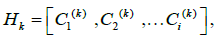(1)

where i=1, 2,…, M ,k=1, 2, …, K.

Here, H k represents the hopping pattern assigned to a particular user k. Further, M and K denote the number of complementary codes, and the number of users, respectively. Note that K can be greater than M. The complementary code set for a particular user is represented as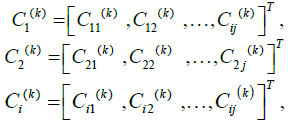(2)

Where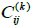represent the jth element code of the ith complementary code used by user k.

In a conventional CDMA system, each user uses a unique spreading code to spread its information symbols, and hence there is no collision among users if the spreading codes are orthogonal. The orthogonally of each complementary code,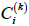in CHMA is based on a flock of element codes,, instead of a single code used in a conventional CDMA system. The Processing Gain (PG) of a particular CHMA system using OCCs is the product of flock size and the number of complementary codes. Each user data is hopped based on the assigned set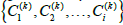of complementary codes during spreading with the information symbols based on the hopping pattern assigned . The spreading data matrix for a particular user k is given by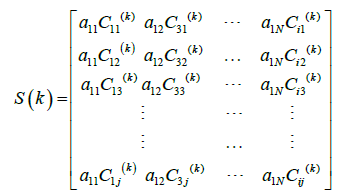(3)

Where a k=[ak1ak2…a k N] represents the data corresponding to user k.

The information transmission using multiple carriers provides ideal Auto-Correlation Function (ACF) and Cross-Correlation Function (CCF) behaviors. In spite of the occurrence of many side lobes during auto-correlation between individual element codes, the sum of autocorrelation between different channels is zero for any time shift, and is denoted as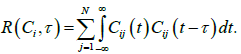(4)

Similarly, the sum of cross-correlation between element codes for different users of the same subcarrier is zero for all possible time shifts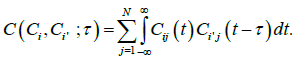(5)

After code hopping, the signals are carrier modulated and transmitted over the fading channel. The transmitted signal over a downlink channel comprising K users with M sets of complementary codes is given by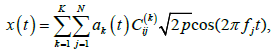(6)

Whereis the jth element code of a particular OCC, which is varied based on the hopping pattern assigned to a particular user. Here, N represents the number of element codes in a particular OCC and p denotes the signal power. Further, ak represent the information bit of a particular user, f j represents the jth subcarrier, The spreaded data using element codes of a particular OCC are sent to the receiver using different subcarriers. The subcarriers required for transmission is based on the set size of element codes.

Receiver section of CHMA with diversity

At the receiver, the received multipath signal at the demodulator is given by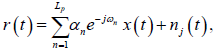(7)

Where n j (t) is the Additive white Gaussian Noise (AWGN) with double sided power spectral density N0/2 experienced by the jth element code? It is assumed to be statistically independent of the fading amplitudes {αn } n α in each channel. Here, L p represents the number of multipaths taken by the signal to reach the receiver.

The received multipath signal is mixed down to baseband and despread using the element codes of a particular user k to produce the information sequence at the correlator output as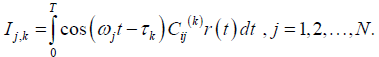(8)

The correlator outputs corresponding to each and every element code are added to give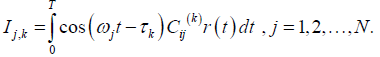(8.1)

The outputs from the L p branch matched filter are individually weighted and then combined by a RAKE-MRC combiner to obtain multipath diversity gain. With MRC, the L p branches are weighted by their respective fading amplitudes  given by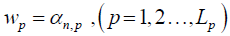(8.2)

The decision variable for the kth user is obtained after combining the individually weighted L p branch signals.

#### Performance of CHMA in a Rayleigh Fading Channel Using OCC

We consider a downlink CHMA communication system under multipath channel conditions where the signal from the Base station (BS) is transmitted to K simultaneous active mobile users employing antenna diversity. Each user employs M orthogonal complementary codes and Binary Phase Shift Keying (BPSK) is used. All the element codes are assumed to experience flat, but correlated fading.

In a complementary coded CHMA system, the Probability Density Function (PDF) of the random variable y in receiving a symbol is given by Equation (17) of  as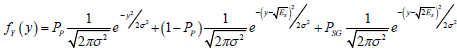,     (9)

Where PP, P SG represent the perforation and synergy probabilities, respectively, given by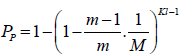(10)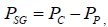(11)

Where M, K, m, l denote the number of OCCs, the number of users, the number of symbol locations in data modulation, and number of multipath components, respectively. Further, σ 2 represents the noise variance denoted as N0/2 where N0 is the single-sided noise spectral density. For BPSK, m=2. Here, Pc represents the code collision probability given by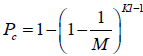(12)

The error probability occurring during transmission of symbol 1 is given by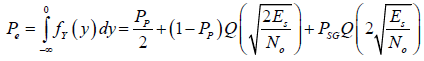(13)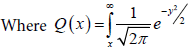The PDF of square of the Rayleigh faded random variable, r, which is γ is given by Equation (5.2.3) on page 314 of  as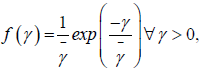(14)

Where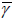is the average received SNR given by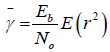(14.1)

Where E denotes the statistical expectation (mean). Here, E (r2) is the average value of (r2), and γ represents the received instantaneous SNR at the input of the receiver due to fading and is given by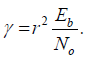(14.2)

The probability of error in a CHMA system over a Rayleigh fading channel using OCCs is obtained by combining (13) and (14) to yield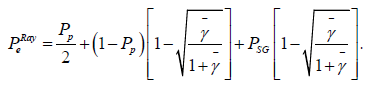(15)

Error probability for a SIMO-CHMA system in a Rayleigh fading channel with MRC

The PDF of a Rayleigh faded random variable γ with independent and identical distribution over L paths with MRC diversity is given by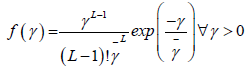(16)

Hererepresents average SNR per channel. The error probability in a SIMO-CHMA system with MRC diversity is obtained by combining (13) and (16) to give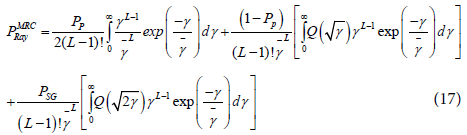The Gaussian probability integral is related to error function as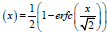. Using the relation between error function and Q(x) with the relation , namely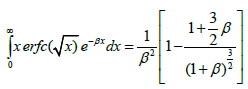(18)

Yields the error probability for dual branch (L=2) MRC over Rayleigh fading channel as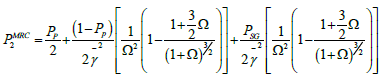(19)

Where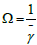. The relation between error function and incomplete gamma function is given by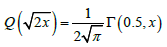(20)

The error probability for L-branch MRC can be obtained by using (20) and equation (6.455/1) of  in (17) to give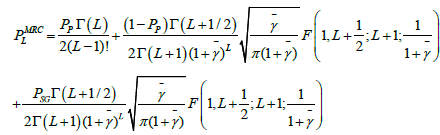(21)

Where F(x, y; z; p) is the Gauss hypergeometric function . Equation (21) can be evaluated using MATLAB.

Error probability of a CHMA system using complementary codes in a Nakagami-m fading channel

In a Nakagami fading channel with parameter m, the PDF of fading channel with nakagami random variable r and instantaneous SNR variable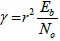given by  as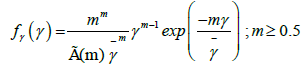(22)

Where= E(R2), and the parameter m is defined as the ratio of moments called the fading figure given by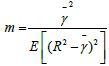Depending on the value of m the Nakagami distribution becomes Rayleigh distribution and when m tends to infinity the distribution approaches an impulse with no fading.

The error probability for CHMA in a Nakagami-m fading channel is obtained by combining (19) and (22), to yield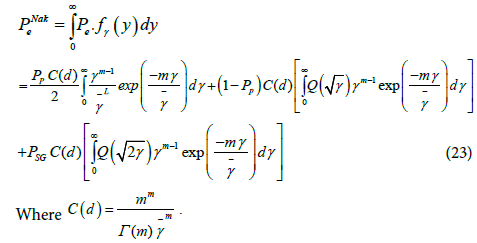The relation between error function and probability integral is given by ,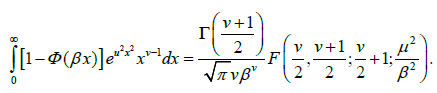Substituting Equation (3.381/4) of  and (21) in (23), the error probability for CHMA system using OCC under Nakagami-m fading channel is given by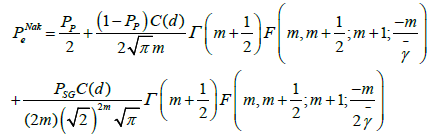(24)

Where F(x, y; z; p) is the Gauss hypergeometric function . Equation (24) can be readily evaluated using MATLAB.

Error probability of a CHMA system using complementary codes in a Nakagami-m fading channel with MRC diversity

The PDF of instantaneous fading amplitude γ with MRC under Nakagami-m fading in each branch is given by [24-27]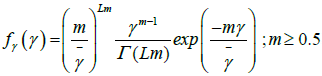(25)

The error probability for CHMA in a Nakagami-m fading channel with MRC diversity is obtained by combining (24) and (25) and getting (26) to yield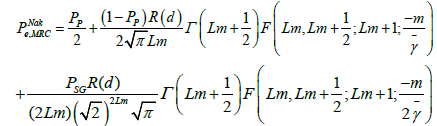(26)

#### Simulation Results

In this paper, the performance of CHMA is simulated using OCC and Gold codes in multipath fading channel for different values of processing Gain and fading conditions. Here BPSK modulation scheme is considered and it was assumed that perfect Channel State Information (CSI) is available at the receiver. Figure 2 shows the analytical BER comparison of SISO and SIMO-CHMA system using OCC under Rayleigh fading channel using (13), with variations in perforation probability. It can be observed that the error probability increases with increase in perforation probability due to the effect of collisions occurring between users using the same code. It can be visualized that when Pp is set to 0.005, the additional required SNR is approximately 4 dB for SIMO-CHMA which increases with increase in perforation probability.

Figure 2: performance comparison of theoretical results obtained for SIMO CHMA system in Rayleigh fading channel for different values of perforation probability.

Figure 3 show the simulated BER performance comparison between SISO and SIMO-CHMA system for different values of (PG), to show the superiority of OCC in multipath channel conditions. It can be observed that for 12 dB SNR the BER for SIMO-CHMA using OCC with PG 32 is 0.0301 which is lower compared to the error of 0.0206 in the case of Gold code with PG 31. In the case of system using OCC with PG 64, the BER 0.0014 which is much lower compared to the BER in SISO-CHMA improvement is two-fold compared to the case with PG 32, where it can be seen that the BER for SIMO is.

Figure 3: performance comparison of BER for SISO and SIMO CHMA system using OCCs in Rayleigh multipath fading channel with variation in processing gain.

In Figures 4 and 5 the performance of SISO and SIMO-CHMA using OCC is compared to Gold codes  to show the superiority of OCC in suppressing both MAI and MPI. The path gain coefficient vectors assumed in both figures are [1 0.8 0.2]. The inter path delay between each path is assumed to be 2 chips. The PG of OCC is 64 and Gold code length is Lc=63. It can be observed that at 8 dB SNR with variations in number of users accessing the system the multipath effect does not degrade the performance of SISO-CHMA system using OCC, but impose a serious threat to the signal detection using Gold codes. It can be further visualized that with increase in number of users, the performance of Gold coded SISO-CHMA deteriorates more rapidly than OCC based CHMA which offers controlled BER with reduced MAI.

Figure 4: BER performance comparison of SISO-CHMA system using OCC and Gold codes in multipath fading channel with variation in users.

Figure 5: BER performance comparison of SIMO-CHMA system using OCC and Gold codes in multipath fading channel using MRC with variations in receiving antennas.

The simulated BER comparison of SIMO in a Multipath fading channel Figure 5 has a higher slope as compared to that of a no diversity technique Figure 4. For a SNR of 4 dB, the MRC diversity with 2 receive antennas provide a BER of 9 × 10-2 for complementary-coded SIMOCHMA, whereas Gold coded SIMO-CHMA has a BER of 8 × 10-1, which is lower compared to the error in a SISO-CHMA. For the no diversity technique Figure 5, the BERs for complementary coded and Gold coded CHMA are 10-1 and 4 × 10-1, respectively. Hence, the use of diversity techniques provides O (10-1) to O (10-2) reduction in BER as compared to the no diversity case with the use of complementary coded CHMA compared to Gold coded CHMA.

#### Conclusions

In this paper, the performance of CHMA using OCCs under Rayleigh and Nakagami-m fading channels with and without diversity combining has been discussed. It is shown that ideal orthogonality of OCCs helps to improve the performance of a CHMA system under existing MAI and multipath interference scenarios. Error probability expressions have been derived and plotted for CHMA with MRC under Rayleigh and Nakagami-m fading channels. We evaluate the system performance separately for OCC and Gold codes by varying the system parameters to verify whether the proposed algorithm accomplishes satisfactory results in multipath channel conditions where orthogonality no longer holds. Simulation results have shown that a CHMA based system using OCCs with diversity offers much higher capacity than a traditional CHMA system which uses Gold codes.

#### References

Citation: Judson D, Bhaskar V, Albert Raj A (2016) Performance of CHMA System Using Orthogonal Complementary Codes under Diversity Incorporated Fading Channels. J Telecommun Syst Manage 5: 136. Doi: 10.4172/2167-0919.1000136

Copyright: ©2016 Judson D, et al. This is an open-access article distributed under the terms of the Creative Commons Attribution License, which permits unrestricted use, distribution, and reproduction in any medium, provided the original author and source are credited.

Select your language of interest to view the total content in your interested language

##### Recommended Journals
Viewmore
###### Article Usage
• Total views: 9743
• [From(publication date): 9-2016 - Aug 17, 2019]
• Breakdown by view type
• HTML page views: 9648
• PDF downloads: 95

## Post your commentCan't read the image? click here to refresh
###### Peer Reviewed Journals

Make the best use of Scientific Research and information from our 700 + peer reviewed, Open Access Journals

International Conferences 2019-20

Meet Inspiring Speakers and Experts at our 3000+ Global Annual Meetings

Top